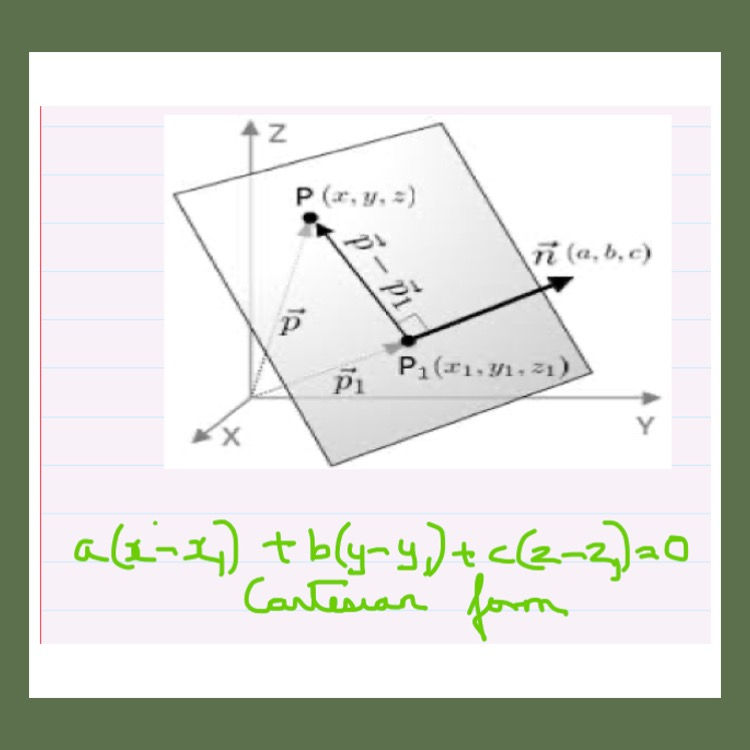top of page
Search

# Solve 3-Dimensional Geometry Questions on Planes Instantly

Updated: Aug 27, 2022

## You'll find the equation of a plane given a point on a plane and the direction ratios of the normal to the plane. These formulas are as shown.Equation of a plane

## Learn how to find the equation of the plane given the foot of the perpendicular from the origin to the plane.## Finding the equation of a plane passing through 2 points and parallel to a line.## Finding the equation of a plane passing through a point and parallel to 2 lines.## Finding the equation of a plane containing 2 lines.## How to check if 3 points are coplanar.## Next, you'll learn about the Intercept form of a plane## 3 D Geometry Problem 13## Check it out here!## Given a plane, how will you find the equation of a plane parallel to this plane. See how to do it here!